Courses

# Physics Test 6 - Modern Physics

## 45 Questions MCQ Test NEET Mock Test Series & Past Year Papers | Physics Test 6 - Modern Physics

Description
This mock test of Physics Test 6 - Modern Physics for NEET helps you for every NEET entrance exam. This contains 45 Multiple Choice Questions for NEET Physics Test 6 - Modern Physics (mcq) to study with solutions a complete question bank. The solved questions answers in this Physics Test 6 - Modern Physics quiz give you a good mix of easy questions and tough questions. NEET students definitely take this Physics Test 6 - Modern Physics exercise for a better result in the exam. You can find other Physics Test 6 - Modern Physics extra questions, long questions & short questions for NEET on EduRev as well by searching above.
QUESTION: 1

Solution:
QUESTION: 2

Solution:
QUESTION: 3

### A point source of light is used in a photoelectric effect.If the source is removed farther from the emitting metal,the stopping potential

Solution:
QUESTION: 4

A proton and an electron are acceleration by the same potential difference Let λ e and   λ p denote the de Broglie wavelengths of the electron and the proton respectively

Solution:
QUESTION: 5

Ionization energy of a hydrogen-like ion A is greater than that of another hydrogen-like ion B. Let r,u,E and L represent the radius of the orbit,speed of the electron, energy of the atom and orbital angular momentum of the electron respectively. In ground state

Solution:
QUESTION: 6
The X-ray beam coming from an X-ray tube
Solution:
QUESTION: 7
Cutoff wavelength of X-rays coming from a Coolidge tube depends on the
Solution:
QUESTION: 8

When an impurity is doped into an intrinsic semiconductor,the cunductivity of the semiconductor

Solution:
QUESTION: 9

Two identical p-n junction may be connected in series with a battery in three ways (figure). The potential difference across the two p-n junctions are equal is: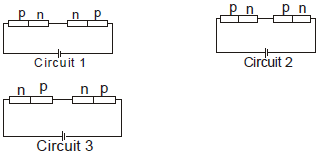Solution:
QUESTION: 10
Two identical capacitors A and B are charged to the same potential V and are connected in two circuits at t= 0 as shown in figure. The charges on the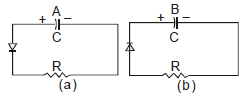capacitors at a time = CR are, respectively,
Solution:
QUESTION: 11
In a transistor
Solution:
QUESTION: 12
A semiconducting device is connected in a series circuit with a battery and a resistance. A current is found to pass through the circuit. If the polarity of the battery is reversed, the current drops to almost zero. The device may be
Solution:
QUESTION: 13

The mass number of a nucleus is

Solution:
QUESTION: 14

Two protons are kept at a separation of 10 nm. Let Fn and  F be the nuclear force and the electromagnetic force between them.

Solution:
QUESTION: 15

Ten grams of 57Co kept in an open containg beta-decays with a half-life of 270 days. The weight of the material inside the container after 540 days will be very nearly

Solution:
QUESTION: 16
A free neutron decays to a proton but a free proton does not decay to a neutron. This is because
Solution:
QUESTION: 17
Magnetic field does not cause deflection in
Solution:
QUESTION: 18
Beta rays emitted by a radioactive material are
Solution:
QUESTION: 19

If elements with principle quantum number n>4 were not allowed in nature, the number of possible elements would be

Solution:
QUESTION: 20

Consider the spectral line resulting from the transition n = 2  → n = 1 in the atoms and ions given below. The shortest wave length is produced by

Solution:
QUESTION: 21

The equation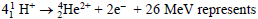Solution:
QUESTION: 22
Fast neutrons can easily be slowed down by
Solution:
QUESTION: 23

Consider α particles, β particles and γ - rays each having an energy of 0.5 MeV. In increasing order of penetrating powers, the radiations are

Solution:
QUESTION: 24

The KX-ray emission line of tungsten occurs at λ  =0.021 nm. The energy difference between K and L levels in this atom is about

Solution:
QUESTION: 25
The electrical conductivity of a semiconductor increases when em radiation of wavelength shorter than 2480 nm is incident on it. The band gap (in eV) for the semiconductor is
Solution:
QUESTION: 26
The dominant mechanisms for motion of charge carriers in forward and reverse biased silicon p-n junctions are
Solution:
QUESTION: 27
As per Bohr model, the minimum energy (in eV) required to remove an electron from the ground state of doubly in ionized Li atom (Z=3) is
Solution:
QUESTION: 28
The circuit shown in the figure contains tow diodes each with a forward resistance of 50 ohms and with infinite backward resistance. If the battery voltage is 6V, the current through the 100 ohm resistance (inAmperes) is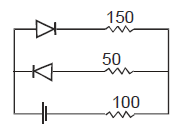Solution:
QUESTION: 29
Which of the following statement is not ture ?
Solution:
QUESTION: 30

A particle of mass M at rest decays into two particles of masses m1 and m2, having nonzero velocities. The ratio of the de Broglie wavelength of the particles λ 1 / λ 2 is

Solution:
QUESTION: 31

Binding energy per nucleon vs. mass number curve for nuclei is shown in the figure W,X,Y and Z are four nuclei indicated on the curve. The process that would release energy is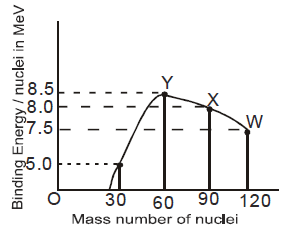Solution:
QUESTION: 32

The transition from the state n = 4 to n =3 in a hydrogen-like atom results in ultraviolet radiation. Infrared radiation will be obtained in the transition

Solution:
QUESTION: 33

A Hydrogen atom and a Li ++ ion are in the second excited state. If lH and  l Li are their respectively electronic angular momenta, and EH and E Li their respectively enrgies, then

Solution:
QUESTION: 34

In a photoelectric experiment anode potenial is plotted against plate current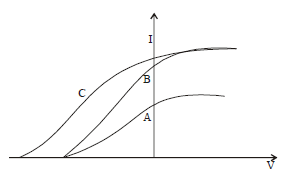Solution:
QUESTION: 35

If a star can convert all the He nuclei completely into oxygen nuclei. The energy released per oxygen nuclei is [Mass of He nucleus is 4.0026 amu and mass of Oxygen nucleus is 15.9994]

Solution:
QUESTION: 36
The largest wavelength in the ultraviolet region of the hydrogen spectrum is 122 nm. The smallest wavelength in the infrared region of the hydrogen spectrum (to the nearrest integer) is
Solution:
QUESTION: 37

Direction : Read the following question and choose
A. If both assertion and reason are true and reason is the correct explantion of assertion
B. If both assertion and reason are true but reason is not the correct explanation of assertion
C. If assertion is true but reason is false
D. If assertion is false but reason is true
Assertion : Nuclear density is almost same for all nuclei
Reason: Size of nucleus  ∞ A 1/3

Solution:
QUESTION: 38
Direction : Read the following question and choose
A. If both assertion and reason are true and reason is the correct explantion of assertion
B. If both assertion and reason are true but reason is not the correct explanation of assertion
C. If assertion is true but reason is false
D. If assertion is false but reason is true
Assertion: A free neutron is not a stable particle
Reason: It decays spontaneously into a proton, an electron and an antineutrino
Solution:
QUESTION: 39
Direction : Read the following question and choose
A. If both assertion and reason are true and reason is the correct explantion of assertion
B. If both assertion and reason are true but reason is not the correct explanation of assertion
C. If assertion is true but reason is false
D. If assertion is false but reason is true
Assertion: In outermost stationary orbit, energy of electron is least negative
Reason: In such an orbit, electron is at maximum distance from the nucleus
Solution:
QUESTION: 40
Direction : Read the following question and choose
A. If both assertion and reason are true and reason is the correct explantion of assertion
B. If both assertion and reason are true but reason is not the correct explanation of assertion
C. If assertion is true but reason is false
D. If assertion is false but reason is true
Assertion: On alpha-decay, group of daughter nucleus shifts
two places to the left from the group of parent nucleus
Reason : An alpha particle carries four units of mass
Solution:
QUESTION: 41
Direction : Read the following question and choose
A. If both assertion and reason are true and reason is the correct explantion of assertion
B. If both assertion and reason are true but reason is not the correct explanation of assertion
C. If assertion is true but reason is false
D. If assertion is false but reason is true
Assertion: Balmer series lies in the visible region of electromagnetic spectrum
Reasion :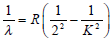where K = 3,4,5....
Solution:
QUESTION: 42
Direction : Read the following question and choose
A. If both assertion and reason are true and reason is the correct explantion of assertion
B. If both assertion and reason are true but reason is not the correct explanation of assertion
C. If assertion is true but reason is false
D. If assertion is false but reason is true
Assertion : X-rays travel with the speed of light
Reason : X-rays are electromagnetic rays
Solution:
QUESTION: 43
Direction : Read the following question and choose
A. If both assertion and reason are true and reason is the correct explantion of assertion
B. If both assertion and reason are true but reason is not the correct explanation of assertion
C. If assertion is true but reason is false
D. If assertion is false but reason is true
Assertion : The conductivity of intrinsic semiconductors at 0 K is zero
Reason: At 0 K no energy is available. No covalent bond can be broken. No free electron is available for conduction
Solution:
QUESTION: 44
Direction : Read the following question and choose
A. If both assertion and reason are true and reason is the correct explantion of assertion
B. If both assertion and reason are true but reason is not the correct explanation of assertion
C. If assertion is true but reason is false
D. If assertion is false but reason is true
Assertion: When base region has larger width, the collector current increases
Reason: Electron hole combination in base results in increase of base current
Solution:
QUESTION: 45
Direction : Read the following question and choose
A. If both assertion and reason are true and reason is the correct explantion of assertion
B. If both assertion and reason are true but reason is not the correct explanation of assertion
C. If assertion is true but reason is false
D. If assertion is false but reason is true
Assertion: A hole is a seat to positive charge
Reason: Hole is created when an electron breaks away from a covalent bond in a semiconductor
Solution: